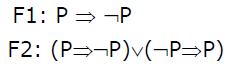# GATE | GATE-CS-2001 | Question 3

Consider two well-formed formulas in prepositional logic.Which of the following statements is correct?

(A) F1 is satisfiable, F2 is valid
(B) F1 unsatisfiable, F2 is satisfiable
(C) F1 is unsatisfiable, F2 is valid
(D) F1 and F2 are both satisfiable

Explanation: The concept behind this solution is:
a) Satisfiable
If there is an assignment of truth values which makes that expression true.
b) UnSatisfiable
If there is no such assignment which makes the expression true
c) Valid
If the expression is Tautology
Here, P => Q is nothing but –P v Q
F1: P => -P = -P v –P = -P
F1 will be true if P is false and F1 will be false when P is true so F1 is Satisfiable
F2: (P => -P) v (-P => P) which is equals to (-P v-P) v (-(-P) v P) = (-P) v (P) =
Tautology
So, F1 is Satisfiable and F2 is valid
Option (a) is correct.

https://en.wikipedia.org/wiki/Tautology_(logic)

This solution is contributed by Anil Saikrishna Devarasetty.

Quiz of this Question

My Personal Notes arrow_drop_up
Article Tags :

Be the First to upvote.

Please write to us at contribute@geeksforgeeks.org to report any issue with the above content.